# 5.7. pyopus.problems.lvns — Lukšan-Vlček set of nonsmooth problems¶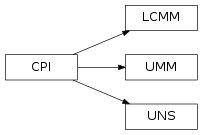Nonsmooth test functions by Lukšan and Vlček (PyOPUS subsystem name: LVNS)

This module is independent of PyOPUS, meaning that it can be taken as is and used as a module in some other package. It depends only on the cpi and the _lvns modules.

The code in the binary module shares variables. Therefore the function should be created and then used immediately. Creating another function may change the previously created one. This sucks, but what can you do? I know! Rewrite the FORTRAN code :)

 [lvns] Lukšan L., Vlček J.: Test Problems for Nonsmooth Unconstrained and Linearly Constrained Optimization, Technical report V-798, ICS AS CR, Prague, 2000.
class pyopus.problems.lvns.UMM(name=None, number=None)

Unconstrained minimax problems from the Lukšan-Vlček test suite.

• name - problem name
• number - problem number (0-24)

Attributes:

• name - problem name
• n - number of variables
• m - number of partial functions
• initial - initial values of variables
• fmin - best known minimum

All test functions in this class are maps from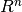to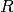. Every function is comprised of m partial functions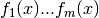where x is a n-dimensional vector.

The actual test function is then constructed as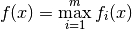or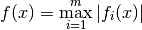cpi()

Returns the common problem interface.

Gradient is not supported. Anyway it is valid only where the functions are smooth.

xmin is also not available.

See the CPI class for more information.

f(x)

Returns the value of the function at x.

fi(x, i=None)

Returns the value of the i-th partial function at x. If i is not given a vector of all partial functions is returned.

names = ['CB2', 'WF', 'SPIRAL', 'EVD52', 'RosenSuzuki', 'Polak6', 'PBC3', 'Bard', 'KowalikOsborne', 'Davidon2', 'OET5', 'OET6', 'GAMMA', 'EXP', 'PBC1', 'EVD61', 'Transformer', 'Filter', 'Wong1', 'Wong2', 'Wong3', 'Polak2', 'Polak3', 'Watson', 'Osborne2']

List of all function names

setup()

Initializes the binary implementation of the function. After this function is called no other function from the same test set may be created or initialized because that will change the internal variables and break the function. Returns an info structure.

class pyopus.problems.lvns.UNS(name=None, number=None)

Unconstrained nonsmooth problems from the Lukšan-Vlček test suite.

• name - problem name
• number - problem number (0-24)

Attributes:

• name - problem name
• n - number of variables
• initial - initial values of variables
• fmin - best known minimum

All test functions in this module are maps fromto. Gradients are valid only where the functions are smooth (continuously differentiable).

cpi()

Returns the common problem interface.

Gradient is not supported because it is untested at this time. Anyway it is valid only where the functions are smooth.

xmin is also not available.

See the CPI class for more information.

f(x)

Returns the value of the function at x.

names = ['Rosenbrock', 'Crescent', 'CB2', 'CB3', 'DEM', 'QL', 'LQ', 'Mifflin1', 'Mifflin2', 'Wolfe', 'RosenSuzuki', 'Shor', 'Colville1', 'HS78', 'ElAttar', 'Maxquad', 'Gill', 'Steiner2', 'Maxq', 'Maxl', 'TR48', 'Goffin', 'MXHILB', 'L1HILB', 'ShellDual']

List of all function names

setup()

Initializes the binary implementation of the function. After this function is called no other function from the same test set may be created or initialized because that will change the internal variables and break the function. Returns an info structure.

class pyopus.problems.lvns.LCMM(name=None, number=None)

Linearly constrained minimax problems from the Lukšan-Vlček test suite.

• name - problem name
• number - problem number (0-14)

Attributes:

• name - problem name
• n - number of variables
• m - number of partial functions
• nc - number of linear constraints
• xl - lower bounds on variables
• xh - upper bounds on variables
• cl - lower bounds on constraint functions
• ch - upper bounds on constraint functions
• Jc - Jacobian of constraints, one row per constraint
• initial - initial values of variables
• fmin - best known minimum

All test functions in this module are maps fromto. Every function is comprised of m partial functionswhere x is a n-dimensional vector.

The actual test function is then constructed asorThe nc constraints are linear and are of the form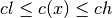Beside linear constraints a problem can also have bounds of the form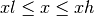c(x)

Returns the values of the constraint functions at x.

cpi()

Returns the common problem interface.

Gradient is not supported. Anyway it is valid only where the functions are smooth.

xmin is also not available.

See the CPI class for more information.

f(x)

Returns the value of the function at x.

fi(x, i=None)

Returns the value of the i-th partial function at x. If i is not given a vector of all partial functions is returned.

names = ['MAD1', 'MAD2', 'MAD4', 'MAD5', 'PENTAGON', 'MAD6', 'EQUIL', 'Wong2', 'Wong3', 'MAD8', 'BPfilter', 'HS114', 'Dembo3', 'Dembo5', 'Dembo7']

List of all function names

setup()

Initializes the binary implementation of the function. After this function is called no other function from the same test set may be created or initialized because that will change the internal variables and break the function. Returns an info structure.

Example file lvns.py in folder demo/problems/

# -*- coding: UTF-8 -*-
# Lukšan-Vlček nonsmooth problems test suites

from pyopus.problems.lvns import *

if __name__=='__main__':
print("Unconstrained minimax problems")
for ii in range(len(UMM.names)):
prob=UMM(number=ii)

# These problems need setting up before they are used.
# Only one problem can be uset at a time.
prob.setup()

print("%2d: %20s n=%2d: f0=%e" % (ii, prob.name, prob.n, prob.f(prob.initial)))
print()

print("Unconstrained nonsmooth problems")
for ii in range(len(UNS.names)):
prob=UNS(number=ii)

# These problems need setting up before they are used.
# Only one problem can be uset at a time.
prob.setup()

print("%2d: %20s n=%2d: f0=%e" % (ii, prob.name, prob.n, prob.f(prob.initial)))
print()

print("Linearly constrained minimax problems")
for ii in range(len(LCMM.names)):
prob=LCMM(number=ii)

# These problems need setting up before they are used.
# Only one problem can be uset at a time.
prob.setup()

print("%2d: %20s n=%2d: f0=%e" % (ii, prob.name, prob.n, prob.f(prob.initial)))
print()